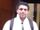## rank scores by data step

To rank scores by data step, If there is a tie between two scores, both should have the same ranking. Note that after a tie, the next ranking number should be the next consecutive integer value. In other words, there should be no "holes" between ranks.

```+----+-------+
| Id | Score |
+----+-------+
| 1  | 3.50  |
| 2  | 3.65  |
| 3  | 4.00  |
| 4  | 3.85  |
| 5  | 4.00  |
| 6  | 3.65  |
+----+-------+```

For example, given the above `Scores` table, your query should generate the following report (order by highest score):

```+-------+------+
| Score | Rank |
+-------+------+
| 4.00  | 1    |
| 4.00  | 1    |
| 3.85  | 2    |
| 3.65  | 3    |
| 3.65  | 3    |
| 3.50  | 4    |
+-------+------+```

1 ACCEPTED SOLUTION

Accepted Solutions

## Re: rank scores by data step

``````data have;input Id  Score ;
cards;
1  3.50
2  3.65
3  4.00
4  3.85
5  4.00
6  3.65
;

proc sort data=have;
by descending score;
run;

data want;
set have;by descending score;
retain rank;
if first.score then rank+1;

run;
``````
Thanks,
Jag
3 REPLIES 3

## Re: rank scores by data step

``````data have;input Id  Score ;
cards;
1  3.50
2  3.65
3  4.00
4  3.85
5  4.00
6  3.65
;

proc sort data=have;
by descending score;
run;

data want;
set have;by descending score;
retain rank;
if first.score then rank+1;

run;
``````
Thanks,
Jagnovinosrin
Tourmaline | Level 20

## Re: rank scores by data step

Hi @Geo- Is it some kind of exclusive datastep challenge? If so, I like it

``````data have;
input Id  Score ;
cards;
1  3.50
2  3.65
3  4.00
4  3.85
5  4.00
6  3.65
;

data _null_;
call symputx('n',n);
set have nobs=n;
stop;
run;

data want;
do n=1 by 1 until(lr);
retain rank score;
set have end=lr;
array t(&n) ;
array k t&n-t1 ;
t(n)=score;
end;
call sortn(of k[*]);
score=t(1);
rank=1;
output;
do n=2 to dim(t);
if t(n) ne t(n-1) then rank+1;
score=t(n);
output;
end;
keep  score rank;
run;
``````novinosrin
Tourmaline | Level 20

## Re: rank scores by data step

``````data have;
input Id  Score ;
cards;
1  3.50
2  3.65
3  4.00
4  3.85
5  4.00
6  3.65
;

data want;
if 0 then set have(rename=(score=_score));
dcl hash H (dataset:'have(rename=(score=_score))',ordered:'d', multidata:'y') ;
h.definekey  ("_score") ;
h.definedata ('_score') ;
h.definedone () ;
dcl hiter i('h');
do while(i.next()=0);
if _score ne score then rank+1;
score=_score;
output;
end;
stop;
keep rank score;
run;``````
Discussion stats
• 3 replies
• 5115 views
• 5 likes
• 3 in conversation